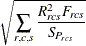# The MDS Procedure

### Formulas

The following notation is used: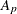intercept for partition p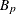slope for partition p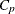power for partition p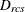distance computed from the model between objects r and c for subject s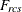data weight for objects r and c for subject s obtained from the cth WEIGHT variable, or 1 if there is no WEIGHT statement

f

value of the FIT= option

N

number of objects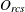observed dissimilarity between objects r and c for subject s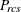partition index for objects r and c for subject s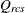dissimilarity after applying any applicable estimated transformation for objects r and c for subject s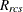residual for objects r and c for subject s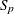standardization factor for partition p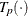estimated transformation for partition p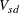coefficient for subject s on dimension d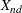coordinate for object n on dimension d

Summations are taken over nonmissing values.

Distances are computed from the model as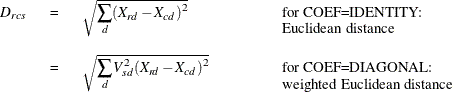Partition indexes are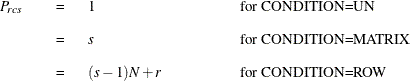The estimated transformation for each partition is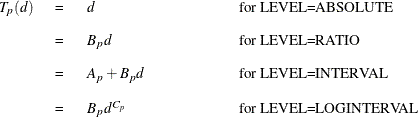For LEVEL=ORDINAL,is computed as a least-squares monotone transformation.

For LEVEL=ABSOLUTE, RATIO, or INTERVAL, the residuals are computed as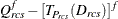For LEVEL=ORDINAL, the residuals are computed as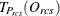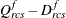If f is 0, then natural logarithms are used in place of the fth powers.

For each partition, let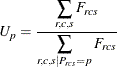and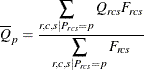Then the standardization factor for each partition is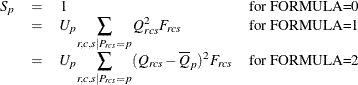The badness-of-fit criterion that the MDS procedure tries to minimize is